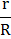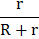# A cell of internal resistanceis connected to a load of resistance. Energy is dissipated in the load, but some thermal energy is also wasted in the cell. The efficiency of such an arrangement is found from the expressionWhich of the following gives the efficiency in this case? a)b)c)d)## Question ID - 150587 :- A cell of internal resistanceis connected to a load of resistance. Energy is dissipated in the load, but some thermal energy is also wasted in the cell. The efficiency of such an arrangement is found from the expressionWhich of the following gives the efficiency in this case? a)b)c)d)3537

(d)

Assuming currentflows through the circuitEnergy dissipated in loadEnergy dissipated in the compete circuitThe efficiencyRelation between resistance, mass and cross-sectional areaNext Question :

 When the forward bias voltage of a diode is changed from 0.6 V to 0.7 V, the current changes from 5 mA to 15 mA. Then its forward bias resistance is a) 0.01b) 0.1c) 10d) 100